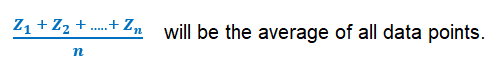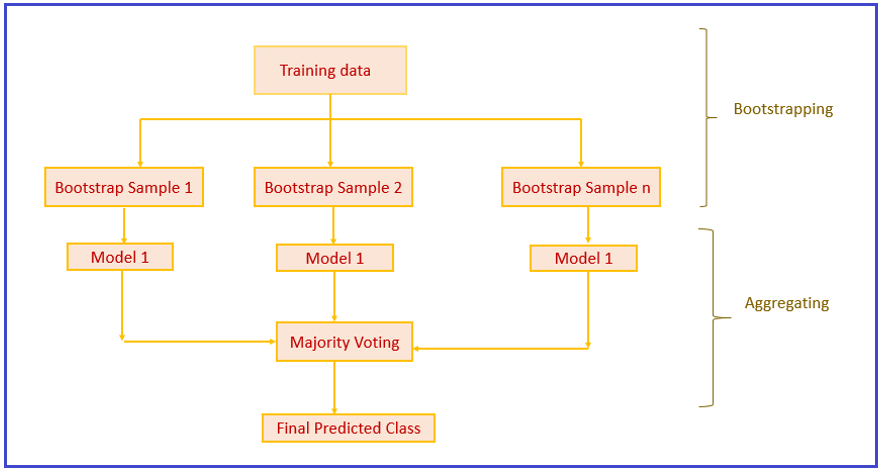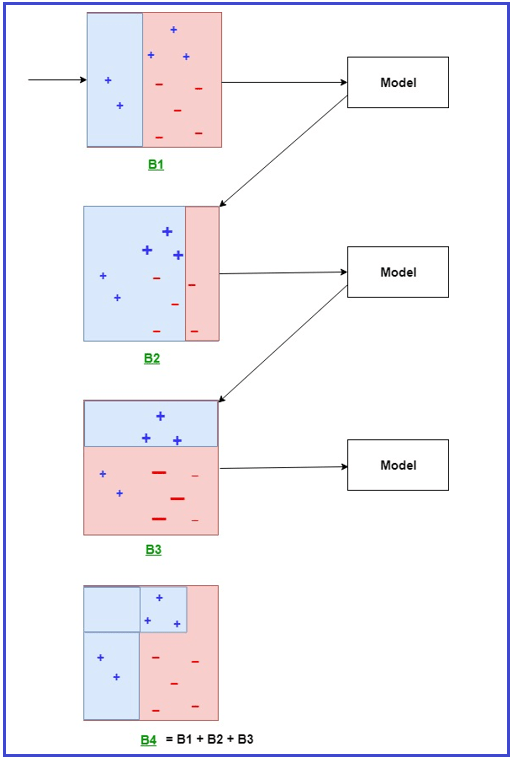# ETS Models in Machine Learning

## ETS Models in Machine Learning

In this article, I am going to discuss ETS Models in Machine Learning with Examples. Please read our previous article where we discussed Time Series Data in Machine Learning with Case Study.

##### Working and Implementation of Different ETS Models in Machine Learning

The ETS models are a group of time series models that have an underlying State Space Model that includes a level component, a trend component (T), a seasonal component (S), and an error term (E).

The ETS models –

1. are not stationary or static.
2. smooth using exponentials
3. use this model if the data has a trend and/or seasonality, as it explicitly models these components.

The ETS model, which stands for Error-Trend-Seasonality, is a time series decomposition model. It divides the series into three parts: error, trend, and seasonality. When dealing with time-series data, it is a univariate forecasting model. It focuses on seasonal and trend elements. Trend techniques model, exponential smoothing, and ETS decomposition are some of the principles included in this model.

Using the three important variables of error, trend, and seasonality aids in the creation of a model to suit the data. These terms will be used in the ETS model for “smoothing.”

For understanding the trend and seasonality of time series data, ETS models can be quite beneficial. The python library ‘statsmodels’ makes plotting the graph and analyzing the data easier.

##### Power of Ensembles

Individuals who operate in groups are more likely to perform well than those who work alone. One advantage of groups is that they may approach a task from a variety of viewpoints, something an individual might not be able to do. A coworker might notice an issue that we were unaware of. The benefits of grouping also apply to machine learning models. By grouping individual weak learners, we can develop a robust, highly accurate model. Ensemble methods are used in the field of machine learning to combine base estimators (i.e. weak learners). Averaging (e.g. bagging) and boosting are two forms of ensemble approaches (eg. gradient boosting, AdaBoost).

Ensemble approaches improve performance while simultaneously lowering the risk of overfitting. Consider a person who is assessing a product’s performance. One person may place too much emphasis on a single feature or detail and hence fail to deliver a well-rounded assessment. When a group of people evaluates a product, each person may concentrate on a different feature or detail. As a result, we limit the risk of focusing too much on a single characteristic. Finally, we’ll have a more generalized assessment. Ensemble approaches, on the other hand, produce a well-generalized model and thereby limit the risk of overfitting.

##### Bagging –

Bagging is the process of combining the predictions of numerous weak learners into a single prediction. We can conceive of it as putting together a group of weak learners at the same time. The total forecast is based on the average of multiple weak learners’ predictions. Random forests are the most frequent algorithm that employs the bagging approach.

##### Boosting –

Boosting is the process of putting together multiple weak learners in a row. We end up with a strong learner from a group of weak learners who are connected in a sequential manner. Gradient boosted decision tree is one of the most widely used ensembles learning algorithms that involve boosting (GBDT). Weak learners (or base estimators) in GBDT are decision trees, just like in random forests.

##### Bagging Decision Trees

High-variance models are decision trees. It means that even a small modification in the training data will result in a completely different model. Overfitting is a common problem with decision trees. An ensemble approach — bagging — can be employed to overcome this problem.

Bootstrap Aggregation is referred to as “bagging.” Bagging entails creating many models using a subset of the data and then aggregating the predictions of the various models to reduce variation.

Assume we have a collection of n independent observations, Z1, Z2,….., Zn. Individual observations have a variance of a2.Similarly, the mean-variance will be a2/n. As a result, increasing the number of data points reduces the variation of the mean. Bagging-decision trees are based on this principle. If we train multiple decision trees in parallel with subsets of data and then later aggregate the results, the variance will get reduced.

Bagging has two steps –

1. Bootstrapping
2. Aggregation
##### Bootstrapping –

We know that by averaging the set of observations, the variance will be decreased. However, we only have one training set and must construct numerous decision trees.

Bootstrapping allows various subgroups to be created from the training data. The bootstrapped samples can then be used to create several trees. Bootstrapping will construct several subgroups by randomly selecting repeated data points from the training set — sampling with replacement.

##### Aggregation –

We now have various bootstrap samples and have created ‘B’ decision trees for each of them. The output will then be aggregated. ‘B’ trees predict the output class for each data point in the test set. The final class is determined via a majority vote procedure.Boosting is an ensemble modeling strategy that aims to create a strong classifier out of a large number of weak ones. It is accomplished by constructing a model from a sequence of weak models. To begin, a model is created using the training data. The second model is then created, which attempts to correct the faults in the previous model. This approach is repeated until either the entire training data set is properly predicted or the maximum number of models has been added.

AdaBoost was the first truly successful boosting algorithm created specifically for binary classification. Adaptive Boosting (AdaBoost) is a prominent boosting approach that merges numerous “weak classifiers” into a single “strong classifier.”

##### Algorithm:

1. Set up the dataset and give each data point the same amount of weight.
2. Provide this as input to the model and find the data points that were incorrectly categorized.
3. Increase the weight of the data points that were incorrectly categorized.
4. If the event got the required results
Go to step 5 now
Else
Go to the second step.
5. End

The AdaBoost method is depicted in the diagram above in a relatively straightforward manner.Let’s understand the whole process stepwise –

1. B1 is made up of ten data points of two types: plus(+) and minus(-), five of which are plus(+) and the other five are minus(-), all of which were given equal weight at the start. The first model attempts to identify the data points by producing a vertical separator line, but it incorrectly identifies 3 plus(+) as minus (-).
2. B2 is made up of the 10 data points from the previous model, with the three incorrectly identified pluses(+) being weighted more heavily such that the current model works harder to correctly categorize them. This model generates a vertical separator line that correctly classifies the previously incorrectly classified pluses(+), but it incorrectly classifies three minuses in this try (-).
3. B3 is made up of the 10 data points from the previous model, with the three incorrectly identified minuses(-) being weighted more heavily such that the current model tries harder to correctly categorise them. This model creates a horizontal separator line that correctly classifies the minuses that were previously misclassified (-).
4. In order to develop a good prediction model, B4 integrates B1, B2, and B3.

Because gradient boosting is a greedy method, it can easily overfit a training dataset. Regularization strategies that punish various sections of the algorithm and generally improve the algorithm’s performance by decreasing overfitting can help it.

Allowing trees to be greedily constructed from subsamples of the training dataset was a major breakthrough in bagging ensembles and random forests.

In gradient boosting models, this benefit can be employed to lower the correlation between the trees in the sequence. Stochastic gradient boosting is the name for this type of boosting.

A subsample of the training data is randomly selected (without replacement) from the whole training dataset at each cycle. The randomly selected subsample is then utilized to fit the base learner instead of the entire sample.

There are a number of different types of stochastic boosting that can be used:

1. Before making each tree, sample rows.
2. Before you make each tree, subsample the columns.
3. Before considering each split, subsample columns.

Aggressive subsampling, such as selecting only half of the data, has been demonstrated to be helpful in general.

In the next article, I am going to discuss Model Tuning Techniques in Machine Learning with Examples. Here, in this article, I try to explain ETS Models in Machine Learning with Examples. I hope you enjoy this ETS Model in Machine Learning with Examples article.